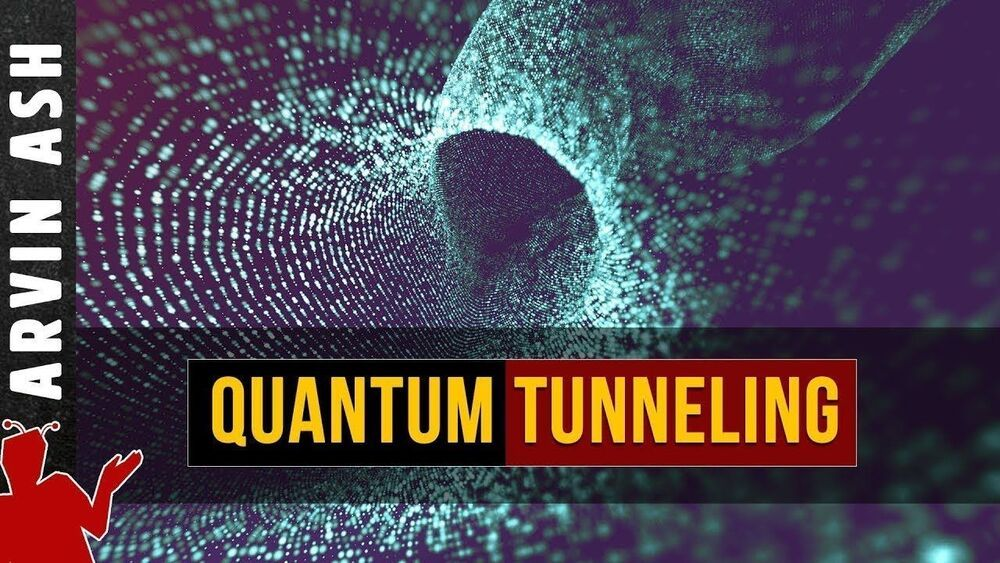## BlogGet MagellanTV here: https://try.magellantv.com/arvinash and get an exclusive offer for our viewers: an extended, month-long trial, FREE. MagellanTV has the largest and best collection of Science content anywhere, including Space, Physics, Technology, Nature, Mind and Body, and a growing collection of 4K. This new streaming service has 3000 great documentaries. Check out our personal recommendation and MagellanTV’s exclusive playlists: https://www.magellantv.com/genres/science-and-tech.

Become a Patron: https://www.patreon.com/bePatron?u=17543985

What is this mysterious quantum tunneling effect, where does it come from? And why is it one of the most important phenomena in physics?

Quantum mechanics shows that quantum objects have a wave-particle duality. What we think of as an electron particle actually behaves like a wave, a probability wave. This means that its position is not a precise location in space. It is defined by a wave function that can only tell us the probability of finding it a particular location when measured. The wave function of a particle exists in all of space, in the entire universe up to infinity. So there is always a non-zero probability of finding the electron anywhere, including outside a barrier.

We can attribute this behavior to the Heisenberg uncertainty principle. It states that the uncertainty in a particle’s position times the uncertainty in its momentum has to be greater than a finite number. Practically this means we cannot know with 100% certainty what the position of that electron is. And the wave function of the electron, which gives us the probability of finding it at any location can be found using the Schrodinger equation.

This equation was developed by Erwin Schrödinger in 1926, and it is the equation that describes the wave nature of matter. The Greek letter psi in the equation is the wave function. The wave function depends on both time and position. It can be both positive or negative, but the square is always positive. The square of the wave function as a function of position is the probability of finding the particle at that position. The Schrödinger equation is a statement of conservation of energy. It says that kinetic energy plus potential energy equals the total energy—But instead of just energies, we have energy operators acting on the wave function of the particle.

For a particle inside a box with a wall of finite thickness and height, we can solve the wave function inside the box, in the barrier and also outside the barrier. We find that the amplitude is non-zero within and outside the barrier. So, it can has some probability of being outside the box.